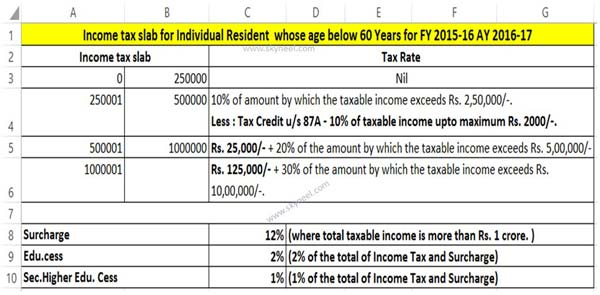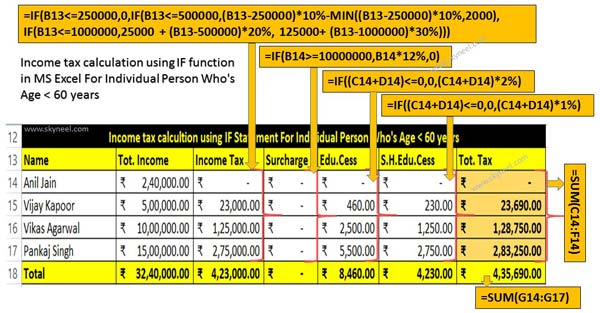# Quick Excel Income tax Calculator for FY 2015-16 AY 2016-17

Quick excel income tax calculator for FY 2015-16 AY 2016-17. compute tax liability for individual resident whose age is below than 60 years.

The budget for the F.Y. 2015-16 A.Y. 2016-17 is already announced by the budget minister. The income tax slab is remain un-changed in this financial year. This article provide quick excel income tax calculator for FY 2015-16 AY 2016-17 for individual resident whose age is below than 60 years i.e. born on or after 1st April 1956.

Income tax is a direct tax which is levied by the Government. Direct tax are levied on the different source of incomes of individuals or different organisations.

## Quick Excel Income tax Calculator

With the help of quick Excel Income tax Calculator for FY 2015-16 AY 2016-17 you can easily compute tax liability for an individual. Income tax is levied on different sources of incomes like salaries, investment, interest etc. from individuals. This article helps you to easily and quickly compute your tax liabilities for salaried individuals with the help of Nested IF function in MS Excel. Nested IF function is used to check more than one extra situations in Microsoft Excel.

If you feel difficulty to compute the income tax then this article helps you to quickly compute your tax liability for salaried individuals whose age is below than 60 years.

For eg. If you want to compute tax liability for salaried individual for FY 2015-16 AY 2016-17. The slab detail for individual resident is given below. Income tax slab for Individual resident who’s aged below 60 years for FY 2015-16 AY 2016-17 (i.e. born on or after 1st April 1956)

 S.No. Income Slabs Tax Rates (Individual resident age below 60 years) 1. Where the taxable income up to Rs. 2,50,000/-. Nil 2. Where the taxable income is greater than Rs. 2,50,000/- but does not exceed Rs. 5,00,000/-. 10% of amount by which the taxable income exceeds Rs. 2,50,000/-. Less : Tax Credit u/s 87A – 10% of taxable income upto maximum Rs. 2000/-. 3. Where the taxable income is greater than Rs. 5,00,000/- but does not exceed Rs. 10,00,000/-. Rs. 25,000/- + 20% of the amount by which the taxable income exceeds Rs. 5,00,000/-. 4. Where the taxable income is greter than Rs. 10,00,000/-. Rs. 125,000/- + 30% of the amount by which the taxable income exceeds Rs. 10,00,000/-.

Note:

1. Surcharge :Surcharge is 12% of the Income Tax, where total taxable income is more than Rs. 1 crore.
2. Education Cess :3% of the total of Income Tax and Surcharge.

Before start computing process of income tax first we have to know about the IF and Nested-IF function in Microsoft Excel, have a look:

IF Function in MS Excel

The IF function is a most popular logical function which is used in the Microsoft Excel. The IF function allows you to quickly evaluate a situation which has two possible outcomes and calculate a different value for each outcome. Sometimes you need to work with situations where there are more than two possible outcomes then you have to use “Nested-IF” function in Microsoft Excel. You can use more than one IF statements is Nested IF function in MS-Excel.

Syntax IF function:

IF (logic test, value if true, value if false)

The logic test compare between given two values by using any comparison operator. The body part of this function are also known as arguments.

Step 1: Open an existing table or create a new table to compute the income tax for individual resident for men or women whose age is below 60 years.Step 2: To calculate the income tax take the cursor on cell C13 and type the following formula to quick compute income tax for FY 2015-16 AY 2016-17:=IF(B13<=250000,0,IF(B13<=500000,(B13-250000)*10%-MIN((B13-250000)*10%,2000), IF(B13<=1000000,25000 + (B13-500000)*20%, 125000+ (B13-1000000)*30%))) and press enter to get the result.

Step 3: To calculate the surcharge on income tax take cursor on cell D13 and type the following formula: =IF(B13>=10000000,B13*12%,0) and press enter to get the result.

Note: Surcharge is applicable when your total income is greater than or equal to 10000000, otherwise it will be zero.

Step 4: Education cess is applicable on total tax liability i.e. (Income tax+Surcharge). To calculate the education cess you have to type the following formula in cell E13:

=IF((C13+D13)<=0,0,(C13+D13)*2%) and press enter to get the result.

Step 5: Secondary higher education cess is also computed on total tax liability i.e. (Income tax+Surcharge). To calculate the sec. higher education cess you have to type the following formula in cell F13:

=IF((C13+D13)<=0,0,(C13+D13)*1%) and press enter to get the result.

Step 6: To compute total tax liability of an individual resident person whose age is below than 60 years then type the following formula in cell G13:

=SUM(C13:F13) and press enter to get the final tax amount.

Note: If you to compute other person income tax liability whose age is below than 60 years then drag the formula of range C13:G13 to C16:G16. Quick Excel Income tax Calculator allows you to easily compute income tax liability for all those men or women whose age in below than 60 years in India.

I hope this Excel Income tax Calculator for FY 2015-16 AY 2016-17 article will be helpful for all those individual residents who want to compute his income tax liability to file his income tax return. If you have any suggestion regarding this article then write us in the comment box. Thanks to all.

#### One Comment

1. hi,
sir, i have read your post for tax calculation on senior citizen and below 60 age also.
but can you make a formula for both in one cell only….with investment minus if any in other cell..